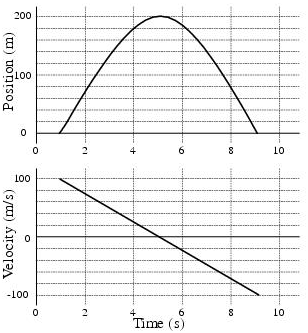# Problem: a) The object's acceleration is:b) The object maintains a zero velocityc) When a clock reads 4 seconds a cart’s velocity in the positive x direction is 6 m/s. When the clock reads 6 seconds, the cart’s velocity in the positive x-direction is 10 m/s. What is the magnitude (in m/s2) of the acceleration of the cart?

###### FREE Expert Solution

This problem involves position, velocity, and time. We'll need to know the relationship between the variables.

79% (419 ratings)###### Problem Detailsa) The object's acceleration is:

b) The object maintains a zero velocity

c) When a clock reads 4 seconds a cart’s velocity in the positive x direction is 6 m/s. When the clock reads 6 seconds, the cart’s velocity in the positive x-direction is 10 m/s. What is the magnitude (in m/s2) of the acceleration of the cart?